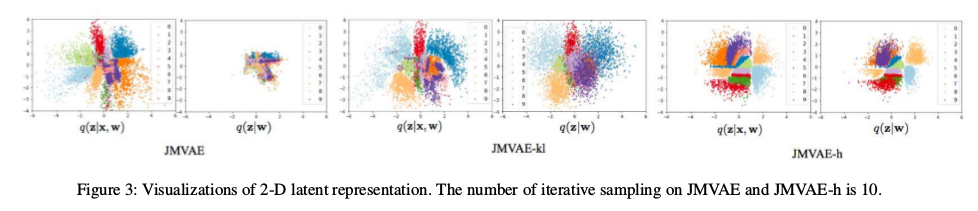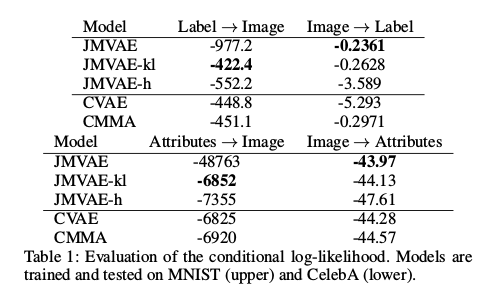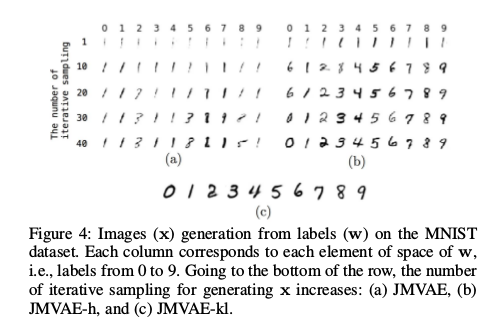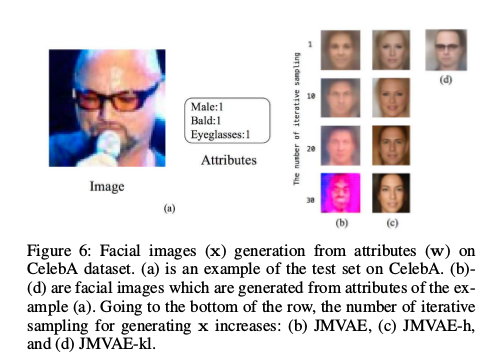VAE在图像和数据的生成上有着不错的效果，对于单模态数据而言VAE可以不错的重构出图像（虽然有些模糊），但是对于双模态或者是更多模态下的利用VAE 则是困难的，JMVAE可以用于双模态的相互生成但是对于模态间维度差异很大或者是一个模态下缺失数据的情况下效果则是不乐观，这篇论文就是在JMVAE 的基础上为了克服模态下缺失数据造成的生成不理想提出了JMVAE-h和JMVAE-kl两种模型用于改进。

# 论文引入

• 提出了两个模型，JMVAE-kl和JMVAE-h，它们可以有效防止潜在变量在缺少高维模态时崩溃
• 模型的双向生成能力与单向VAE类似
• 模型可以适当获得包含不同模态信息的联合表示，可做双向生成

# JMVAE、JMVAE-h、JMVAE-kl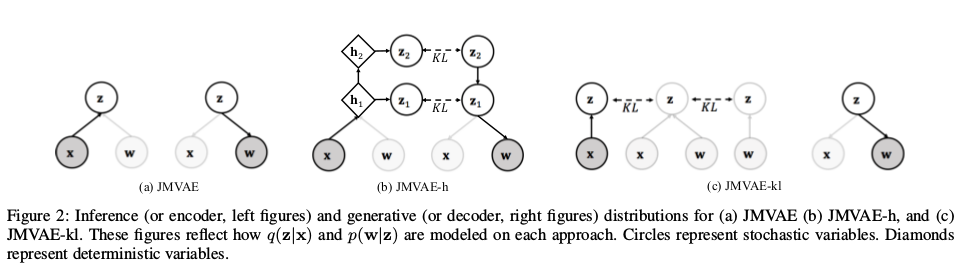JMVAE：

$\mathcal L_{JM}(x,w) = -D_{KL}(q_\phi (z \vert x,w) \Vert p(z)) + E_{q_\phi (z \vert x,w)}[log p_{\theta_x}(x \vert z)] + E_{q_\phi (z \vert x,w)}[log p_{\theta_w}(w\vert z)]$

JMVAE-h：

JMVAE-h是在JMVAE的基础上对潜在变量z对其做分层，使其具有层次结构。假设对因变量分为了L层$z_1,…,z_L$，此时JMVAE的联合表示$p(x,w)$ 可以表示为$p(x,w) = \int … \int p_{\theta}(z_L)p_{\theta}(z_{L-1}\vert z_L)…p_{\theta}(x\vert z_1)p_{\theta}(w\vert z_1)dz_1…dz_L$ 其中所有条件分布$p_{\theta}(z_{l-1}\vert z_l)$都是高斯分布。对于隐变量分布$q_{\phi}(z_1,…,z_L \vert x,w)=q_{phi}(z_1 \vert x,w)…q_{phi}(z_L \vert x,w)$， 其中所有条件分布$q_{phi}(z_l \vert x,w)$都是高斯分布。通俗点说，$x,w$编码得到隐变量$z_1$，$z_1$再通过神经网络做变换（分层）到$z_2$ 这样一直下去直到第L层，文中定义$L=2$。此时的损失函数为：

\begin{aligned} \mathcal L_{JM_h}(x,w) = -\sum_{l=1}^L E_{q_\phi (z_{l+1} \vert x,w)}D_{KL}(q_\phi (z_l \vert x,w) \Vert p_{\theta}(z_l \vert z_{l+1})) \\ + E_{q_\phi (z_1 \vert x,w)}[log p_{\theta_x}(x \vert z_1)] + E_{q_\phi (z_1 \vert x,w)}[log p_{\theta_w}(w\vert z_1)] \end{aligned}

JMVAE-kl：

JMVAE-h虽然较好的解决模态缺失问题但是迭代采样是耗时的，尤其是处理高维数据的时候，如果我们已经有了单模态下编码得到的隐变量$q_{\lambda}(z \vert x)$， 另一个模态的隐变量$q_{\lambda}(z \vert w)$，对于联合分布$q_\phi (z \vert x,w)$，将单模态的隐变量和联合分布一一拉近，最后的联合分布将是包含两个模态编码信息的隐变量。 但是由于单独训练了单模态的编码器，所以解码过程出的数据质量相对要好。

$\mathcal L_{JM_kl}(x,w) = \mathcal L_{JM}(x,w) - [D_{KL}(q_\phi (z \vert x,w) \Vert q_{\lambda}(z \vert x)) + D_{KL}(q_\phi (z \vert x,w) \Vert q_{\lambda}(z \vert w))]$

JMVAE-kl需要单独训练编码器，相比代价高一点，但是生成的质量要高一点，后面实验部分也有说明。

# 模型对比